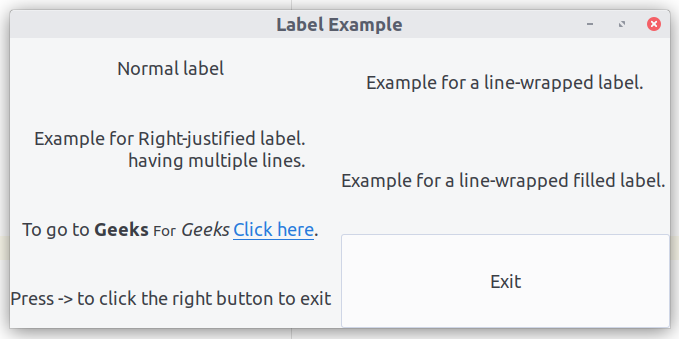Related Articles
Label in Python GTK+ 3
• Last Updated : 22 Jun, 2020

Labels are the main method of placing non-editable text in windows. For example, label can be used as a title placed next to entry widget. Some of the important features of the label are –

• The selection of labels can be done using `Gtk.Label.set_selectable()`. Selectable labels allow the user to copy the contents to the clipboard. Labels contain useful information to copied such as error messages that can be made as a selectable label.
• Label text justification and word-wrapping are possible by the methods `Gtk.Label.set_justify()`, `Gtk.Label.set_line_wrap()` respectively.
• Also, `Gtk.Label` supports clickable hyperlinks. The markup for links is borrowed from HTML, using the a with `href` and title attributes. GTK+ renders links similar to the way they appear in web browsers, with colored, underlined text.
• Mnemonics are the underlined characters in the label, used for keyboard navigation; can be created by giving a string with an underscore before the mnemonic character, such as “_Geeks For Geeks” to the function `Gtk.Label.set_text_with_mnemonic()`.

To create a label follow the below steps:

1. import GTK+ 3 module.
2. Create main window.
3. Create a Box.
4. Implement Label.
5. Implement Button.
Example :
 `import` `gi`` ` `gi.require_version(``"Gtk"``, ``"3.0"``)``from` `gi.repository ``import` `Gtk`` ` `  ` `class` `LabelWindow(Gtk.Window):``     ` `    ``def` `__init__(``self``):``        ``Gtk.Window.__init__(``self``, title ``=``"Label Example"``)``         ` `        ``# Create Box``        ``hbox ``=` `Gtk.Box(spacing ``=` `10``)``        ``hbox.set_homogeneous(``False``)``        ``vbox_left ``=` `Gtk.Box(orientation ``=` `Gtk.Orientation.VERTICAL, ``                    ``spacing ``=` `10``)``        ``vbox_left.set_homogeneous(``False``)``        ``vbox_right ``=` `Gtk.Box(orientation ``=` `Gtk.Orientation.VERTICAL, ``                    ``spacing ``=` `10``)``        ``vbox_right.set_homogeneous(``False``)``  ` `        ``hbox.pack_start(vbox_left, ``True``, ``True``, ``0``)``        ``hbox.pack_start(vbox_right, ``True``, ``True``, ``0``)``         ` `        ``# Create label``        ``label ``=` `Gtk.Label(``"Normal label"``)``        ``vbox_left.pack_start(label, ``True``, ``True``, ``0``)``         ` `        ``# Create justified label``        ``# with multiple lines``        ``label ``=` `Gtk.Label(``"Example for "``                ``"Right-justified label.\n"``                ``"having multiple lines."``)``        ``label.set_justify(Gtk.Justification.RIGHT)``        ``vbox_left.pack_start(label, ``True``, ``True``, ``0``)``  ` `        ``label ``=` `Gtk.Label(``            ``"Example for a line-wrapped label."``  ` `        ``)``        ``label.set_line_wrap(``True``)``        ``vbox_right.pack_start(label, ``True``, ``True``, ``0``)``  ` `        ``label ``=` `Gtk.Label(``            ``"Example for a line-wrapped filled label. "``        ``)``        ``label.set_line_wrap(``True``)``        ``label.set_justify(Gtk.Justification.FILL)``        ``vbox_right.pack_start(label, ``True``, ``True``, ``0``)``         ` `        ``# Create label markup``        ``label ``=` `Gtk.Label()``        ``label.set_markup(``            ``"To go to Geeks "``            ``"For Geeks "``            ``'Click here.'``        ``)``        ``label.set_line_wrap(``True``)``        ``vbox_left.pack_start(label, ``True``, ``True``, ``0``)``         ` `        ``# Create label mnemonic``        ``label ``=` `Gtk.Label.new_with_mnemonic(``            ``"_Press -> to click the right button to exit"``        ``)``        ``vbox_left.pack_start(label, ``True``, ``True``, ``0``)``        ``label.set_selectable(``True``)``         ` `        ``# Create Button``        ``button ``=` `Gtk.Button(label ``=``"Exit"``)``        ``label.set_mnemonic_widget(button)``        ``vbox_right.pack_start(button, ``True``, ``True``, ``0``)``  ` `        ``self``.add(hbox)``  ` `  ` `window ``=` `LabelWindow()``window.connect(``"destroy"``, Gtk.main_quit)`` ` `# Display the window.``window.show_all()`` ` `# Start the GTK + processing loop``Gtk.main()`

Output :Attention geek! Strengthen your foundations with the Python Programming Foundation Course and learn the basics.

To begin with, your interview preparations Enhance your Data Structures concepts with the Python DS Course. And to begin with your Machine Learning Journey, join the Machine Learning – Basic Level Course

My Personal Notes arrow_drop_up